# Weak solution

(diff) ← Older revision | Latest revision (diff) | Newer revision → (diff)

of a differential equationin a domainA locally integrable functionsatisfying the equation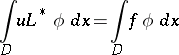for all smooth functions(say, of class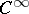) with compact support in. Here, the coefficients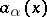in

are assumed to be sufficiently smooth and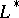stands for the formal Lagrange adjoint of: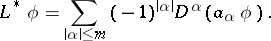For example, the generalized derivative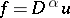can be defined as the locally integrable functionsuch thatis a weak solution of the equation.

In considering weak solutions of , the following problem arises: under what conditions are they strong solutions (cf. Strong solution)? For example, in the case of elliptic equations, every weak solution is strong.

How to Cite This Entry:
Weak solution. Encyclopedia of Mathematics. URL: http://encyclopediaofmath.org/index.php?title=Weak_solution&oldid=12915
This article was adapted from an original article by A.P. Soldatov (originator), which appeared in Encyclopedia of Mathematics - ISBN 1402006098. See original article